# AP Physics 1 Multiple-Choice Practice Test 19

### Test Information10 questions18 minutes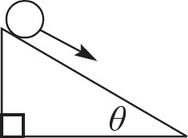1. If a ball is rolling down an inclined plane without slipping, which force is responsible for causing its rotation?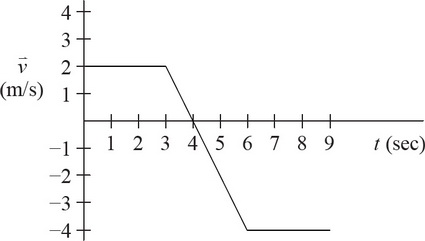2. The graph above shows the velocity of an object as a function of time. What is the net displacement of the object over the time shown?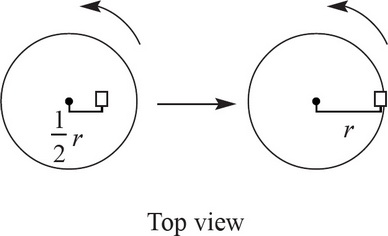3. An object is resting on a platform that rotates at a constant speed. At first, it is a distance of half the platform's radius from the center. If the object is moved to the edge of the platform, what happens to the centripetal force that it experiences? Assume the platform continues rotating at the same speed.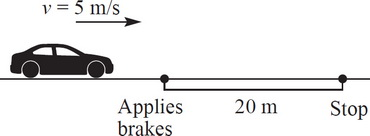4. A car of mass 1000 kg is traveling at a speed of 5 m/s. The driver applies the breaks, generating a constant friction force, and skids for a distance of 20 m before coming to a complete stop. Given this information, what is the coefficient of friction between the car's tires and the ground?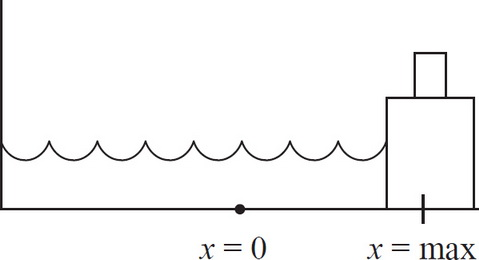5. A spring-block system is oscillating without friction on a horizontal surface. If a second block of equal mass were placed on top of the original block at a time when the spring is at maximum compression, which of the following quantities would NOT be affected? Assume that the top block stays on the bottom block.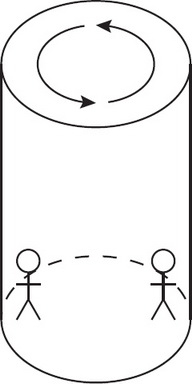6. A certain theme park ride involves people standing against the walls of a cylindrical room that rotates at a rapid pace, making them stick to the walls without needing support from the ground. Once the ride achieves its maximum speed, the floor drops out from under the riders, but the circular motion holds them in place. Which of the following factors could make this ride dangerous for some riders but not others?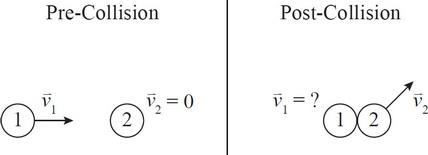7. Two balls collide as shown above. Given the final direction of the second ball's motion after the collision, which of the following is a possible direction for the first ball to move after the collision?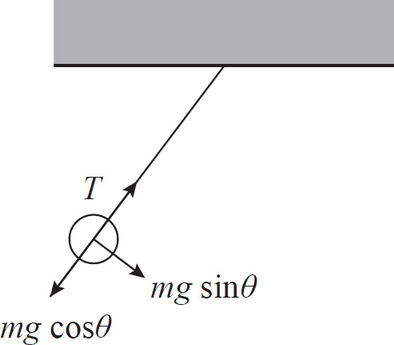8. As a pendulum swings back and forth, it is affected by two forces: gravity and tension in the string. Splitting gravity into component vectors, as shown above, produces mg sinθ (the restoring force) and mg cosθ. Which of the following correctly describes the relationship between the magnitudes of tension in the string and mg cosθ?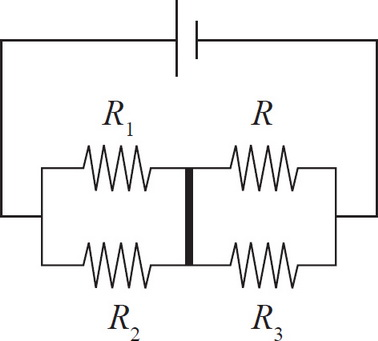9. In order to ensure that no current will pass through the cross path (bold in the image above), what must the resistance of R be in terms of R1, R2, and R3 ?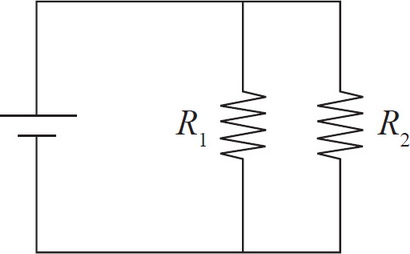10. The circuit above has two resistors in parallel. The first, R1, will have its resistance steadily increased. The second, R2, will have a constant resistance of R2 = 4 Ω. Which of the following graphs correctly depicts the total resistance of the circuit, RT, as a function of R1?